# How many ways can we classify polygons?

### Definition

A polygon is a closed geometric figure whose sides are simple line segments. Each corner of a polygon where two sides intersect is called a vertex of the polygon.

For example, a triangle is a polygon with 3 sides. There are also three vertices, one at each point. This is the simplest polygon, because you can't construct one with just 1 or 2 sides (try it!).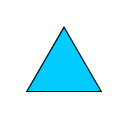### Classification of Polygons

A polygon may be identified by the number of sides.

(1) A polygon with 4 sides is called a quadrilateral.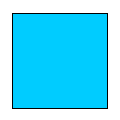(2) A polygon of 5 sides is called a pentagon.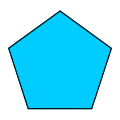A polygon of 8 sides is called an octagon.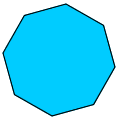A polygon of 10 sides is called a decagon.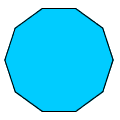A polygon of 12 sides is called a dodecagon.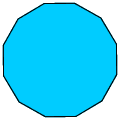NOTE: There are many more polygons, but the ones listed here are some of the most popular and most often taught in geometry classes. Polygons with more than 12 sides are usually referred to as n-gons. As in, a polygon with 56 sides is a 56-gon.

### Other terms

In an equiangular polygon, each angle has the same degree measure. A square is an example of an equiangular polygon because each of the 4 angles form 90 degrees. The same can be said about a rectangle.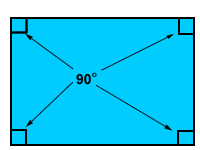In an equilateral polygon, each side has the same length. In triangle ABC below, all sides are 12 feet making triangle ABC equilateral.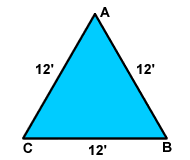A regular polygon is BOTH equiangular and equilateral. A square is a regular polygon because all sides have the same length and all angles measure the same: 90 degrees.

### Classification of Triangles

Triangles may be classified by

(A) their sides, or

(B) their angles

A scalene triangle has 3 different length sides.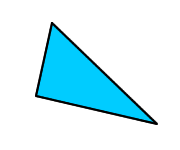An isosceles triangle has two equal sides and one side that is not equal.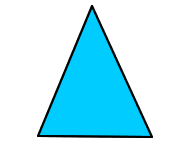An equilateral triangle has 3 equal sides.In an acute triangle, all of the angles will measure less than 90 degrees.A right triangle will always have one 90-degree angle.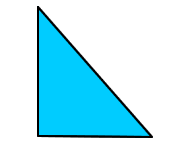An obtuse triangle will always have one angle whose measure is greater than 90 degrees but at the same time less than 180 degrees.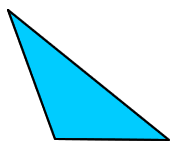### Median and Altitude (Height)

A median of a triangle is a segment drawn from a vertex of the triangle to the midpoint of the opposite side.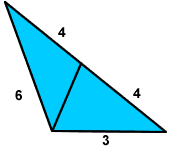An altitude or height of a triangle is a segment drawn from a vertex of the triangle perpendicular to the opposite side or to the opposite side extended.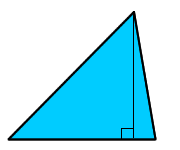### Distance

The word distance in geometry is always understood as the shortest path. The distance between a point and a line is the length of the perpendicular segment drawn from a point that is not on the line to a point on the line. The distance from A to line BC below is 6, because that is the length of the altitude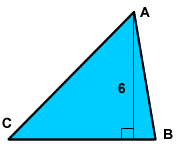Lesson provided by Mr. Feliz